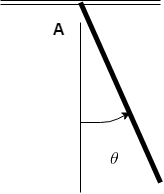# The rod has mass m and length l. A pin through end A of the rod slides without friction in the...

## Question:

The rod has mass m and length l. A pin through end A of the rod slides without friction in the horizontal track shown. The rod is released from rest in the position {eq}\theta= 90^{\circ} {/eq} . For the position {eq}\theta= 0 {/eq} , find

(a) the velocity of the center of mass,

(b) the velocity of end A,

(c) the vertical reaction force that the pin at A exerts on the rod.## Torque.

The torque is the force which provides body twisting or the turning movement around a fixed axis is termed as torque. The rotational equilibrium is achieved when the net torque on the body gives a value equal to zero.

Become a Study.com member to unlock this answer! Create your account

(a)

The formula for torque on system.

{eq}\tau = mg \times \dfrac{L}{2}\sin \theta {/eq}

Here, the length of the rod is {eq}L {/eq}.

Su...Torque: Definition, Equation & Formula

from

Chapter 4 / Lesson 11
565K

You've probably heard of torque before, perhaps while discussing cars. Now learn what it really is and what it has to do with rotational equilibrium. Then, work through an example problem that combines the two.